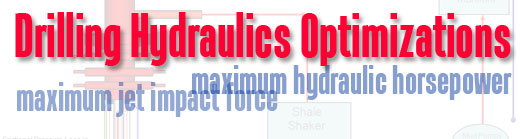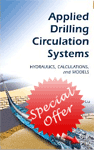# Drilling Hydraulics Optimization

For drilling hydraulics optimization, there are two ways which is the maximum jet impact force and the maximum hydraulic horsepower used to indicate good hole cleaning.The Maximum Jet Impact Force

The concept of this method is based on the concept that the best condition to clean the well is when the force provided by the jets is the maximum. Based on the mathematical solution, this condition is occurred when bit nozzles and circulation rate are properly selected to create pressure drop across the bit which is equal to 48% of total pump pressure.

For example, if the maximum expected pressure is 3,000 psi, you need to select the flow rate and the bit nozzles which will generate 1,440 psi (48% of 3,000 psi) pressure drop across the bit and leave 1560 psi (52% of 3,000 psi) for the system pressure loss.

The Maximum Hydraulic Horsepower

The concept of this method is based on the concept that the best condition to clean the well is when the hydraulic horsepower at the bit is the maximum. Based on the mathematical solution, this condition is occurred when bit nozzles and circulation rate are properly selected to create pressure drop across the bit which is equal to 65% of total pump pressure.

For example, if the maximum expected pressure is 3,000 psi, you need to select the flow rate and the bit nozzles which will generate 1,950 psi (65% of 3,000 psi) pressure drop across the bit and leave 1050 psi (35% of 3,000 psi) for the system pressure loss.

What are the criteria to select the drilling hydraulics optimization methods?

In shallow, large diameter sections where the rate of penetration and cutting volume is high, you need higher flow rate to clean the wellbore. With these criteria, the maximum impact force method is recommended because it gives you 19.5% higher flow rate than the maximum hydraulic horsepower.

In deeper, smaller diameter sections where the ROP is lower than the shallow section and cuttings affecting down force become a drilling hydraulic issue. Hence, you need higher jet velocity and pressure at the bottom hole. To match the requirement, the best optimization way for this case is the maximum hydraulic horsepower because it gives 14.3% higher jet velocity and 34.7% higher pressure than the maximum impact force.

Reference:Drilling Hydraulic Books

Share the joy